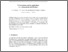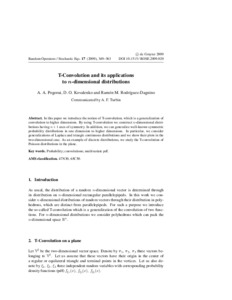# T-Convolution and its applications to n-dimensional distributions

Pogoruі, А. А. and Kovalenкo, D. О. and Rodríguez-Dagnіno, Ramón М. (2009) T-Convolution and its applications to n-dimensional distributions. Stochastic Eqs (17). pp. 349-363.Preview
Text

## Abstract

In this paper we introduce the notion of T-convolution, which is a generalization of convolution to higher dimensions. By using T-convolution we construct n-dimensional distributions having n+1 axes of symmetry. In addition, we can generalize well-known symmetric probability distributions in one dimension to higher dimensions. In particular, we consider generalizations of Laplace and triangle continuous distributions and we show their plots in the two-dimensional case. As an example of discrete distributions, we study the T-convolution of Poisson distributions in the plane.

Item Type: Article Q Science > QA Mathematics > Mathematical Analysis Faculty of Physics and Mathematics > Department of Mathematical Analysis Ірина Ігорівна Таргонська 21 Oct 2014 09:12 15 Aug 2015 09:11 http://eprints.zu.edu.ua/id/eprint/13248View Item